# Inertia forces and crankshaft torque

In a previous tutorial we examined action and reaction forces generated in the elements of a slider and crank mechanism by using simple free body diagrams for an engine and a compressor.  In this tutorial we expand free body diagrams to include inertia forces and inertia torques generated in the individual elements (slider, connecting rod and crank arm), a general topic known as kinetics.  We also consider how to calculate the torque produced at the crankshaft of an engine delivering power through a crank mechanism.

#### Overview

The following free body diagrams illustrate the concepts of inertia forces and torques.

(note: the terms inertia torque and inertia moment are synonymous)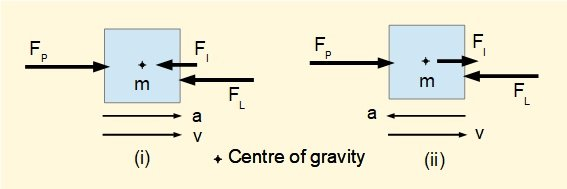In diagram (i)  FP is the force which must be applied to accelerate mass m at a m/s2 against a constant load force FL  (ignoring friction).  The force required to produce this acceleration is Fa = m x a.  We say the inertia of mass m "resists acceleration" through a "pseudo" inertia force  FI which is equal and opposite to Fa and acts through the centre of gravity.  We then use D'Alembert's principle to treat accelerating masses as if they are in static equilibrium by incorporating inertia forces and moments.  Thus in our example with the inertial force  FI  and the sum of forces = 0 ("pseudo" equilibrium condition) gives  FP = FL + FI .  However, the true net force on mass m, Fa  =    FP  -  F(not  in equilibrium because the mass is accelerating).

By contrast, in diagram (ii) force FP must be applied to decelerate mass m against the same constant load force FL  with acceleration =  -a.  The inertia force FI now acts in the opposite direction resisting deceleration. In this case sum of forces = 0 gives  FP = FL - FI.

note: arrows v and  a in the above diagrams illustrate directions of velocity and acceleration vectors.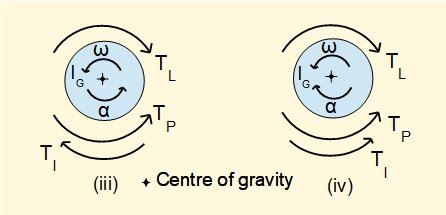Diagrams (iii) and (iv) show the inertia torques TI when torque TP is applied a rotating mass with mass moment of inertia IG  and clockwise rotation against a constant load torque TL  with angular acceleration α  radians/sec2 .  The torque Tα required to produce angular acceleration α   is  IG x α and the reactive inertia torque TI is equal and opposite.   In diagram (iii) (clockwise α ) TP = TL + TI.  .  In diagram (iv) (counterclockwise α) TP = TL - TI .

#### Inertia forces and torques in a slider and crank mechanism

We use of inertia forces and moments to take account of forces in the crank mechanism associated with acceleration of masses.

The basis of this analysis is that inertia forces and moments act through or about the centre of gravity of the moving machine element.  Thus we begin by determining  the mass and centre of gravity of each element which for this example are shown on the diagram below.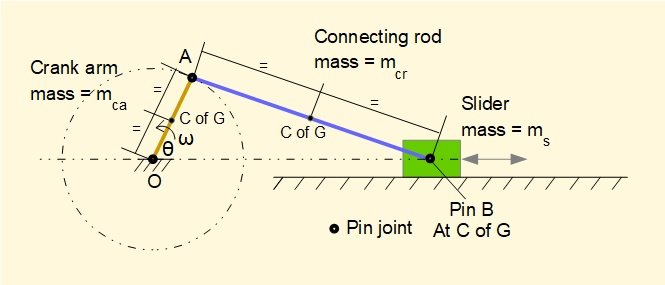For simplicity we assume the crank arm and connecting rod have uniform cross section along their length, thus the centres of gravity are at the mid-points.

Firstly we will identify the inertia forces and moments generated by acceleration of the individual elements and then illustrate with a numerical example.

#### Inertia of the crank arm

In this tutorial we restrict our analysis to a crank arm rotating with constant angular velocity ω, thus the crank arm has no angular acceleration and correspondingly no inertia torque. We take account of the centripetal acceleration of point A directed radially towards centre of rotation O when considering connecting rod AB.

#### Inertia of the slider

We established in an earlier tutorial that the slider experiences linear acceleration which varies constantly throughout the crank cycle. There are four distinct phases in the cycle when the absolute slider velocity is increasing or decreasing.  In the diagram below we show the direction of inertia forces FIs for each phase of the crank cycle.  For a horizontal slider the first phase starts at the inner dead centre position (crank angle ω   = 0°).  The transition between second and third phases occurs at outer dead centre (crank angle ω  = 180°).

In all cases FIs = ms x aB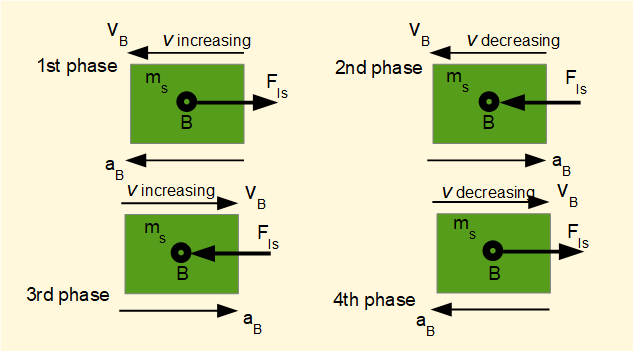#### Inertia of the connecting rod

To determine the inertia forces generated by the mass of the connecting rod we require: (i) the linear acceleration of the centre of gravity (point gAB) relative to point O which we designate agAB, (ii) the inertia moment of AB about the C of G which we designate TIAB  = IgAB where Ig is the mass moment of inertia about the C of G and αAB is the angular acceleration of AB.

To obtain agAB we use the acceleration diagram developed in a previous tutorial shown below.  Note this diagram is specifically for crank angle ω = 50° .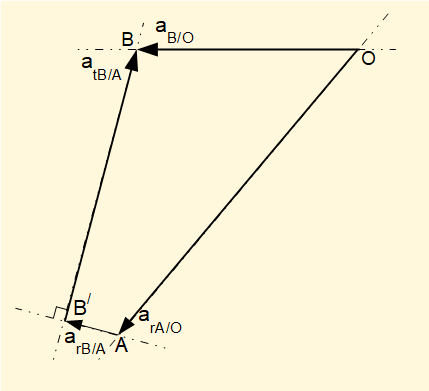To recap, accelerations shown on  this diagram are:

• arA/O the acceleration of point A relative to point O in a radial direction along crank arm AO.  Because angular velocity ω of the crank arm is constant in this analysis there is no tangential component of acceleration of A relative to O.
• arB/A the acceleration of point B relative to point A in a radial direction along connecting rod AB.
• atB/A the acceleration of point B relative to point A tangential to connecting rod AB.
• aB/O the acceleration of point B relative to point O, which is the linear acceleration of the slider mass considered above.

To develop this acceleration diagram to find  agAB (see below) firstly show the acceleration of point B relative to point A, aB/A which is the sum of radial and tangential components.  In our example the acceleration of point gAB is at the mid-point of this line thus line OgAB represents agABThis acceleration is the absolute value relative to ground point O and is opposed by inertia force FIgAB = mcr  x  agAB acting through point gAB. where  mcr is the mass of the connecting rod.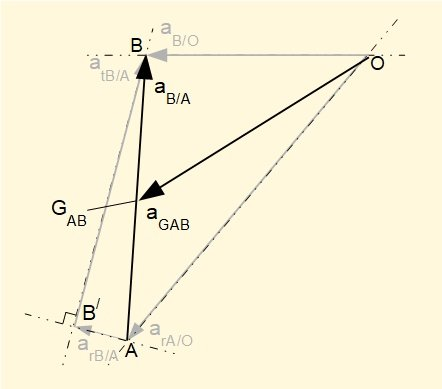To find the inertia moment about point gAB viz. TIAB  = Ig x  αAB  we proceed as follows.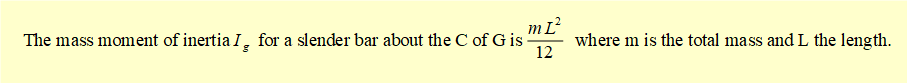αAB = AB x atB/A  is obtained from the acceleration diagram (see the numerical example below).

We illustrate accelerations, inertia force and inertia torque acting on the connecting rod in the diagrams below. Note again that this diagram illustrates the direction of acceleration for crank angle θ = 50° .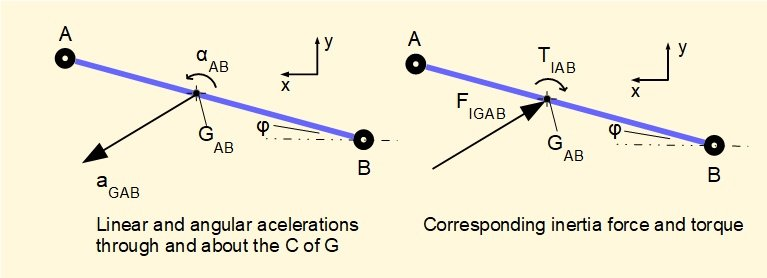The inertia force and torque result in reaction forces at pin joints A and B.  The object of the following numerical example is to find these reaction forces.

#### Example

The physical parameters of the crank mechanism in the example, shown below, are the same as the examples in the kinematics tutorials.  In this idealised arrangement the load torque on the crank arm varies such that the angular velocity ω remains constant through a complete cycle while maintaining a constant force FP on the slider.  We also ignore the effects of friction.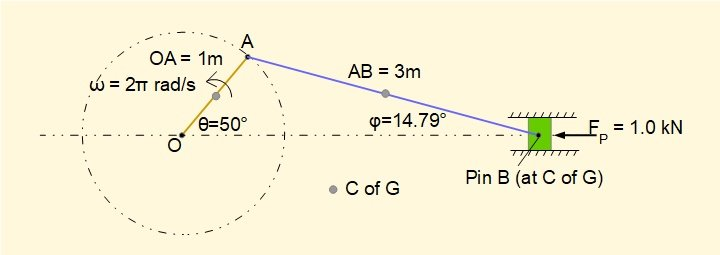Individual elements have the following mass.  The C of G is located at the mid-point of each element.

• Mass of slider (mS) = 10 kg
• Mass of connecting rod (mCR) = 5 kg
• Mass of crank arm  (mCA) = 2 kg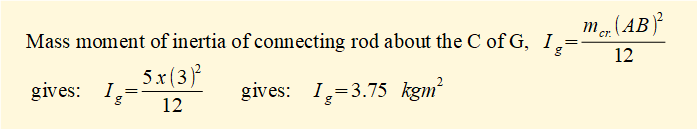Force FP = 1.0 kN  is applied to the slider in the direction shown (for crank angles > 180°  the direction of FP would reverse)

A free body diagram of connecting rod AB is shown below for crank angle  = 50°.  We use sum of forces in x direction = 0, sum of forces in y direction = 0 and moments about C of G =0 to find the unknown reaction forces.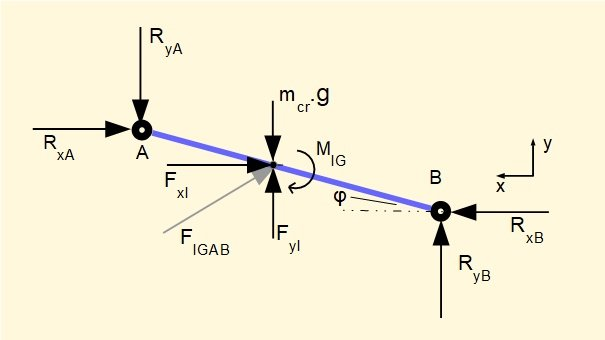RxB is the horizontal reaction force exerted on B by the slider and acts in a +ve direction on the x axis. We reasonably deduce that the horizontal reaction force at point A, RxA , acts in the -ve direction.  We also reasonably deduce that the vertical reaction forces RyB at point B and RyA at point A will be +ve and -ve respectively on account of the direction of thrust along the connecting rod.

MIg is the inertia moment about the C of G.

As a first step we obtain the value of RxB from the free body diagram for the slider (below) where RxB is the equal and opposite reaction force on the slider.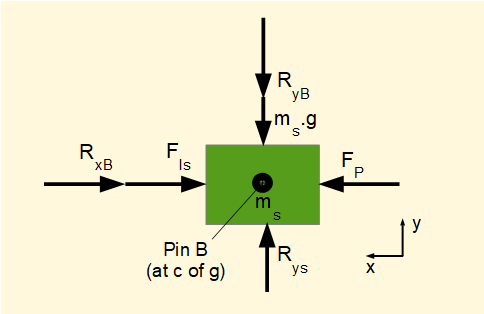The inertia force on slider mass ms, FIs = ms x aB where aB is the linear acceleration of point B.  From a previous tutorial we know for this mechanism and crank angle θ = 50°  that aB = 23.4 m/s2.   Note in passing that reaction force RyB on the slider is opposed by reaction force Rys from the cylinder wall.  This vertical reaction force also opposes gravity force ms.g.

For sum of horizontal forces on the slider = 0:

FP - FIs - RxB = 0        Hence RxB = FP - FIs

Inserting known values gives:     RxB = 1000 - (10  x 23.4) N = 766 N

We now calculate inertia force FIgAB acting on the connecting rod through its C of G.  From the acceleration diagram below derived in a previous tutorial for this mechanism we obtain by geometry the value of agAB and its direction relative to the horizontal axis.Referring to the free body diagram for the connecting rod above the inertia forces through the Cof G are:

FxI = mcr x agAB x cos(31.79°) = 5 x 28.68 x  cos(31.79°) = 121.9 N

FyI = mcr x agAB x sin(31.79°) = 5 x 28.68 x sin(31.79°) = 75.5 N

For sum of horizontal forces on connecting rod = 0:

RxB - FxI - RxA = 0        gives: RxA =   RxB  - FxI     gives: RxA = 766 - 121.9 = 644.1 N

For sum of vertical forces on connecting rod = 0:

FyI - RyA - mcr.g + RyB = 0        gives: RyA - RyB = FyI - mcr.g        gives: RyA - RyB = 75.5 - (5 x 9.81)        gives: RyA - RyB = 26.5 N

For sum of moments about Cof G of connecting rod = 0 (counterclockwise moments +ve):

RyA x 1.5 x cos(14.79°) - RxA x 1.5 x sin(14.79°) + RyB x 1.5 x cos(14.79°) - RxB x 1.5 x sin(14.79°)  - MIg = 0

MIg = Ig x αAB and from a previous tutorial for this mechanism we know αAB = 9.91 rad/sec2

Substitute RyA = 26.5 + RyB

gives: (26.5 + RyB ) x 1.5 x cos(14.79°)  - 644.1 x 1.5 x sin(14.79°) +  RyB  x 1.5 x cos(14.79°) - 766 x 1.5 x sin(14.79°)  - (3.8 x 9.91) = 0

gives: 38.4 + 1.45 x RyB - 246.6 + 1.45 x RyB - 293.3 - 37.7 = 0

gives: 2.9 x RyB = 593.2        gives: RyB = 185.9 N    and     RyA = 185.9 + 26..5 = 212.4 N

From the free body diagram for the slider:

vertical reaction force Rys = RyB + ms.g        gives: Rys = 185.9 + (10 x 9.81) = 284 N

The completed free body diagram for the connecting rod is shown below.  Values are rounded to whole numbers.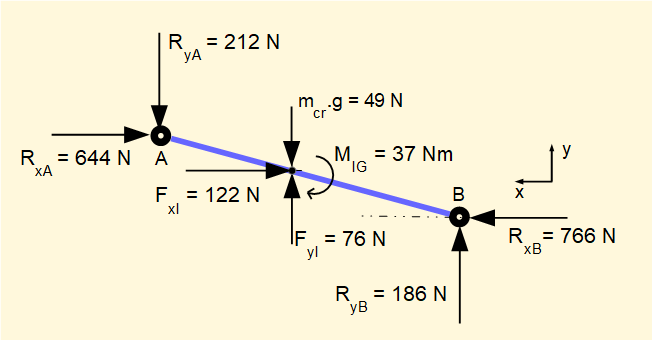It should be emphasised again that inertia moments and forces vary continuously throughout the cycle of the crank mechanism.  This example is a snapshot at crank angle = 50° where inertia forces oppose the driving force and consequently reduce the torque available at the crankshaft.  At other points in the cycle inertia forces aid the driving force and increase the crankshaft torque.  In the next section we look at crankshaft torque in more detail.

#### Crankshaft torque

It is important to know the torque Tca produced by an engine at the crankshaft.  We can obtain crankshaft torque directly from the reaction forces on the crank arm OA at crank pin A which are equal and opposite to the reaction forces RxA and RyA on the connecting rod derived in the previous example.  The free body diagram for the crank arm is shown below. We have ignored the gravity force ( = 10 N) which is insignificant in this instance.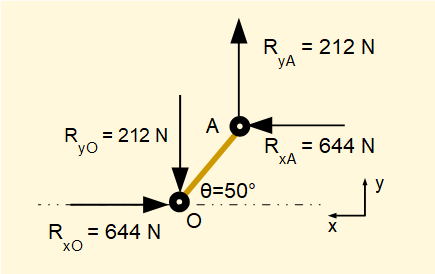Crank arm AO = 1.0 m

Hence crankshaft torque Tca = RxA x 1.0 x  sin(50°) + RyA x 1.0 x cos(50°) = 644 x sin(50°) + 212 x cos(50°) = 626 Nm

Note: the complete free body diagram representing static equilibrium for the crank arm would include a clockwise moment about point O equal in magnitude to Tca.

#### Crankshaft torque - alternative method

Consider the diagram below derived in a previous tutorial showing velocities relative to ground point O of slider B (vB) and crank pin A (vA) and construction of the velocity pole for connecting rod AB.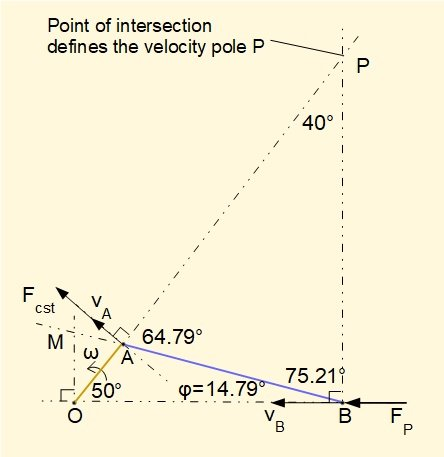We add to this diagram the external horizontal force FP applied to the slider and the resultant force FcaT at crank pin A perpendicular to crank arm OA which delivers torque Tca to the crankshaft such that Tca = FcaT x OA

Using the principle that the rate of work done at point B must equal the rate of work done at point A it follows that: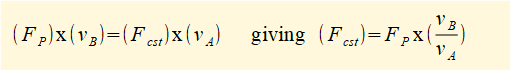From the diagram above, it follows from velocity pole P  that:  vB = PB x ωAB and vA = PA x ωAB  where ωAB is the angular velocity of connecting rod AB.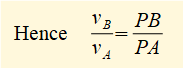Now on the velocity pole diagram extend line BA and take a perpendicular line from point O to intersect extended line BA at point M forming triangle AOM.  Triangles APB and AOM are similar such that: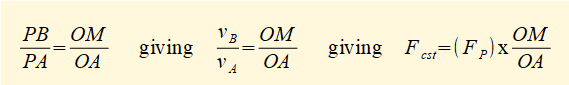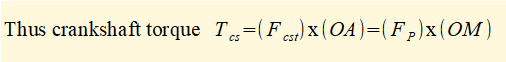In this example OM is calculated from the extended velocity pole diagram above by simple geometry giving the value OM = 0.94 m.

We defined FP as the external force on the slider which in the previous example = 1 kN, giving the crankshaft torque by this method Tca = 1000 x 0.94 = 940 Nm compared to 626 Nm from the previous calculation.

Thus this second method gives a first approximation of crankshaft torque excluding inertia forces and torques for the slider and connecting rod and gravity forces.

A better approximation of crankshaft torque takes account of the inertia force generated by the slider mass.  From the previous example the net horizontal reaction force at B taking account of this inertia force, RxB = 766 N, giving Tca = 766 x 0.94 = 720 Nm.  The remaining discrepancy is accounted for by the inertia force and inertia torque generated by the connecting rod and the gravity force of the rod.

#### Variation of crankshaft torque with crank angle

For convenience we used a crank angle = 50° for the above examples but crankshaft torque varies continuously throughout a full crank cycle. In previous tutorials we developed general expressions for velocities and accelerations of elements in the mechanism.  For crankshaft torque there is a very convenient general expression derived from the extended acceleration diagram (see above) where we noted that: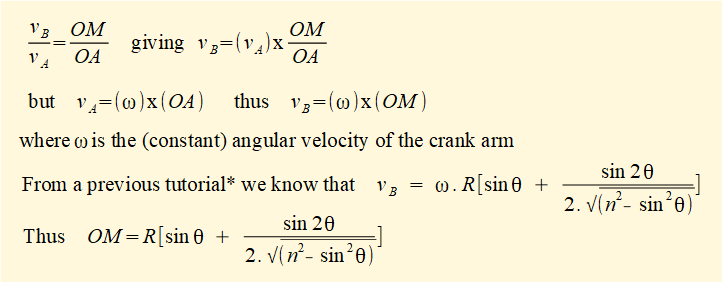* (previous tutorial)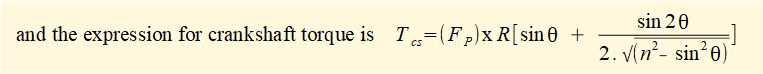The diagram below plots crankshaft torque Tca against crank angle using the above formula for the parameters applied in the previous examples.  The diagram shows a full cycle for crank angle θ  from 0° to 360° with the direction of the external force Fp = 1 kN on the slider reversed at θ = 180°.

The effect of the inertia force generated by acceleration of the slider is illustrated (the inertia force and torque generated by acceleration of the connecting rod is not included).  This clearly shows where the slider inertia force has a positive or negative effect on crankshaft torque.

In a real engine the torque delivered to the load is smoothed by the rotational energy of a flywheel which cyclically transfers rotational energy to and from the crank mechanism resulting in a relatively smooth "mean torque " in continuous operation.  We look at this aspect in another tutorial.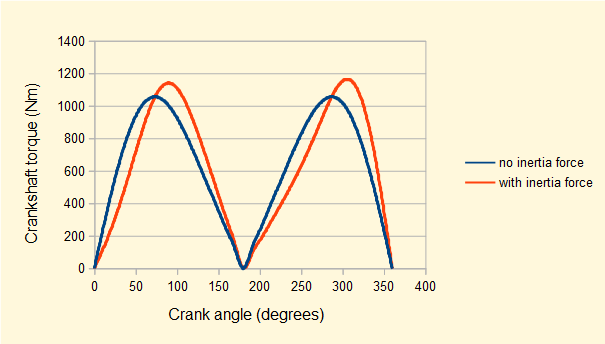I welcome feedback at:

### Tutorials - mechanical vibrations

###### Forced vibrations with damping

Alistair's tutorials 2020